# Power BI Desktop Memory Issue Resolution - Part 2

### Colin Maitland, 08 February 2019

In part one of this article, I described my experience of a “not enough memory to complete this operation” error I encountered in Power BI desktop. In this part, I will describe the cause and resolution for this issue.

The cause of the issue was actually the formula used for generating the Sequential Day Number column. This calculated Column is generated after the Date table is generated. The purpose of this column is to provide a Sequential Day Number for every Date in the Date table in such a way that 29th February, i.e. Leap Year Day, has the same Sequential Day Number as 28th February. This is then used by measures that perform calculations such as ‘Date’[Date] - 365. The following image shows the generated Sequential Day Number for some dates prior to, including, and after 29th February 2016. The Sequential Day Number for 29th February 2016 is the same as that for 28th February 2016.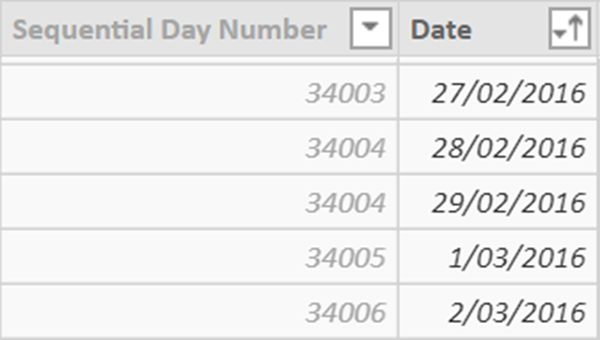This is the formula that was being used. It calculates the Sequential Day Number, starting at 1 for the first Date, by selecting and counting all Dates excluding all occurrences of 29th February prior to and including the Date of the current row being processed. This formula is executed for every row in the Date table; i.e. in my case 35,429 Dates. For each higher Date the number of Dates selected by the formula increases. For 29th February 2016 the formula selects and counts 34,004 Dates.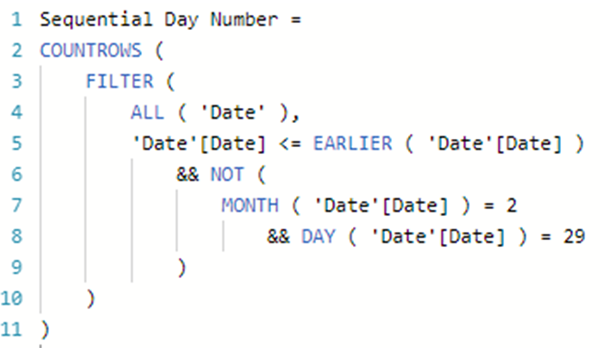However, after the Date table had been regenerated by the CALENDARAUTO() function for a 95-year period the RAM required to calculate the Sequential Day Number column on my computer was exceeding the amount of available 8 GB RAM. As a result of this finding, I realised that having 16 GB of RAM would be more suitable for my needs. However, being limited to only 8 GB RAM I chose to optimise the formula, which is a good thing to do anyway.
This is the optimised formula. This formula calculates the Sequential Day Number, starting at 1 for the first Date, by calculating the current Date’s numeric value, counting and subtracting all Leap Year Days prior to and including the Date of the current row being processed, calculating and subtracting the numeric value of the very first Date in the Date table, and then adding 1 to ensure that sequence starts at 1 rather than at 0. This version of the formula also uses variables for readability, understanding and performance reasons; i.e. ALL ( 'Date'[Date] ) is invoked once rather than twice and EARLIER ( 'Date'[Date] ) is replaced with <= _DATE.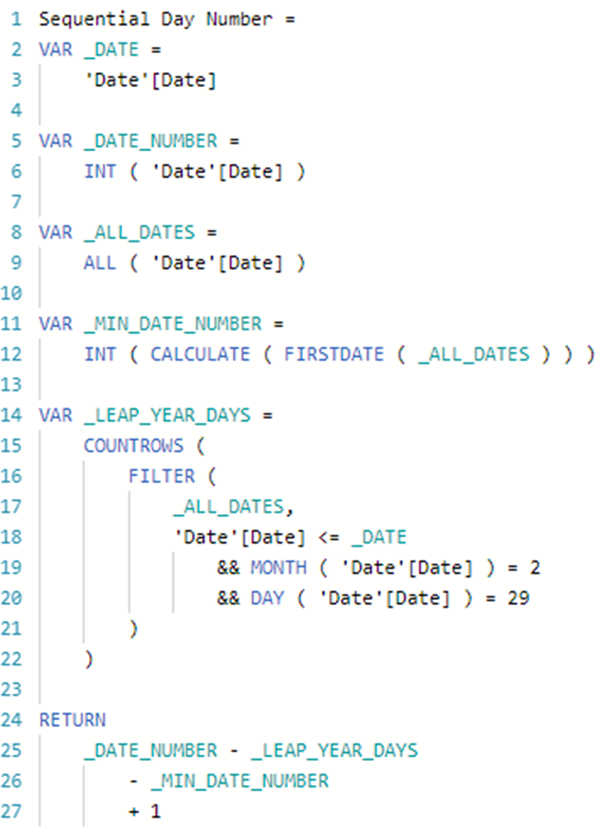For 29th February 2016 the Sequential Day Number is calculated as follows:

•    _DATE = 29/2/2016
•    _DATE_NUMBER = 42,429; i.e. the numeric value for 29/2/2016
•    _MIN_DATE_NUMBER = 8,402; i.e. the numeric value for 1/1/1923
•    _LEAP_YEAR_DAYS = 24
•    _DATE_NUMBER minus _LEAP_YEAR_DAYS minus _MIN_DATE_NUMBER plus 1 = 34,004.

The key difference between these two formulas is that the COUNTROWS() step in the first formula selects and counts all Dates prior to and including the Date being processed excluding Leap Year Days but the second formula only selects and counts the Leap Year Days prior to and including the Date being processed; e.g. for 29th February 2016, the first formula selects 34,004 Dates but the second formula only selects 24 Dates.

The generation of the Sequential Day Number column still takes a couple of minutes on my computer to complete, however. I therefore then also changed the formula used for generating the Date table to use CALENDAR() rather than CALENDARAUTO(). This was appropriate because there is no need to have a Date dimension related to the Date of Birth in this specific Data Model. The Date dimension only needed to accommodate the Date values in the Referrals table, a period of five years currently and only increasing by one year at a time going forward. The following image shows the first few lines of the formula used to generate the Date table.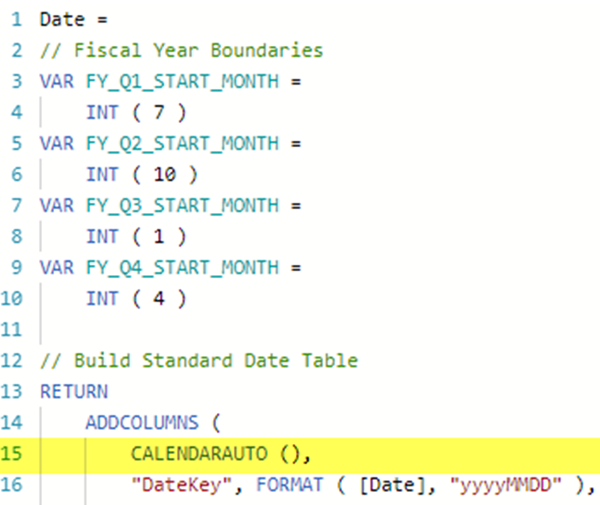I ensured the revised formula accommodated the fact that the Date dimension would need to dynamically include all years for the earliest Date value in the Referral table to the latest Date value in the Referral table and that this would change over time. Therefore, no hard-coding of a Minimum Date or Maximum Date to be used by the CALENDAR() function.

The following image shows the first few lines of the revised formula. It now uses two Measures and the CALENDAR() rather than CALENDARAUTO() function.

For this Data Model, the Minimum Date measure calculates the minimum Date Referred value from the Referrals table and the Maximum Date measure calculates maximum Date value from the combined Date Referred, Date Active, Date Discharged and Date Repeated fields in the Referral table. The Measures exist in a separate to the Date table so that the formula used for generating the Date table remains generic and reusable from deployment to deployment without any need to change it. The only changes that might need to be made from deployment to deployment are those that relate to the formulas used by the Minimum Date and Maximum Date Measures.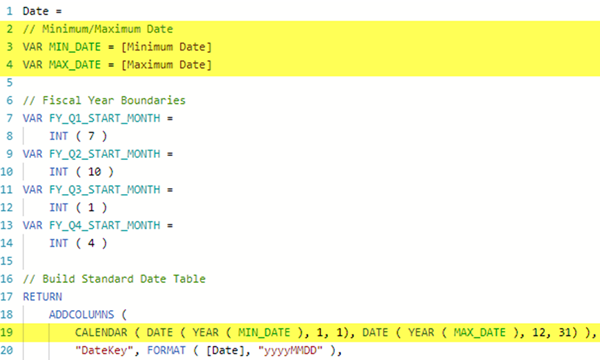In conclusion, the generation of the Date table was optimised in two ways, firstly by optimising the formula used for generating the Sequential Day Number for each Date in the Date table and secondly by optimising the formula used for generating the Date table. The first optimisation is essential and the second is optional.